# Chairs

Determine the number of seats in the seventh row and ninth row, if the 3rd row has 14 seats and in every next row of seats has five more than the previous row.

a7 =  34
a9 =  44

### Step-by-step explanation: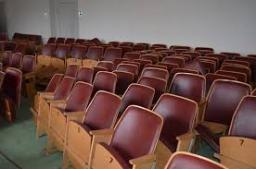Did you find an error or inaccuracy? Feel free to write us. Thank you!Tips to related online calculators
Do you have a linear equation or system of equations and looking for its solution? Or do you have a quadratic equation?

## Related math problems and questions:

• Students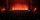In the front row sitting three students and in every other row 11 students more than the previous row. Determine how many students are in the room when the room is 9 lines, and determine how many students are in the seventh row.
• Cinema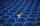How many rows of seats are in the stands, if you know that the first row has 24 seats and the last 50 seats and each subsequent row has two seats more than the row before? How many seats are in the stands?
• SeatsSeats in the sport hall are organized so that each subsequent row has five more seats. First has 10 seats. How many seats are: a) in the eighth row b) in the eighteenth row
• Cinema 4In the cinema are 1656 seats and in the last row are 105 seats, in each next row 3 seats less. How many are the total rows in cinema?
• Cinema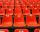Cinema auditorium is built for 3300 people. The first row is planned for 36 seats and each next gradually 4 more. How many rows of seats will have auditorium?
• Determine AP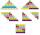Determine the difference of the arithmetic progression if a3 = 7, and a4 + a5 = 71
• Cans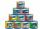How many cans must be put in the bottom row if we want 182 cans arrange in 13 rows above so that each subsequent row has always been one tin less? How many cans will be in the top row?
• Fifth memberDetermine the fifth member of the arithmetic progression, if the sum of the second and fifth members equal to 73, and difference d = 7.
• The HotelThe Holiday Hotel has the same number of rooms on each floor. Rooms are numbered with natural numerals sequentially from the first floor, no number is omitted, and each room has a different number. Three tourists arrived at the hotel. The first one was in
• Table and chairs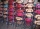Four people should sit at a table in front of a row of 7 chairs. What is the probability that there will be no empty chair between them if people choose their place completely at random?
• HP - harmonic progressionDetermine the 8th term of the harmonic progression 2, 4/3, 1,…
• Volume of woodEvery year, at the same time, an increase in the volume of wood in the forest is measured. The increase is regularly p% compared to the previous year. If in 10 years the volume of wood has increased by 10%, what is the number p?
• Chocholate pyramid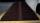How many chocolates are in the third shelf when at the 8th shelf are 41 chocolates in any other shelf is 7 chocolates more the previous shelf.
• Roof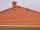Tiles are stacked in rows on the trapezoidal shaped roof. At the ridge is 15 tiles and each subsequent row has one more tile than in the previous row. How many tiled is covered roof if lowermost row has 37 tiles?
• Sum of the seventeen numbersThe sum of the 17 different natural numbers is 154. Determine the sum of the two largest ones.
• Quotient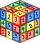Determine the quotient and the second member of the geometric progression where a3=10, a1+a2=-1,6 a1-a2=2,4.
• Unknown number 7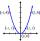Calculate unknown number whose 12th power when divided by the 9th power get a number 27 times greater than the unknown number. Determine the unknown number.